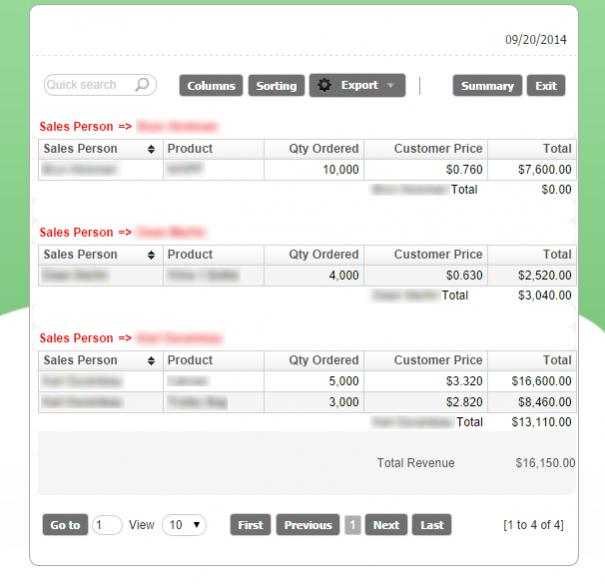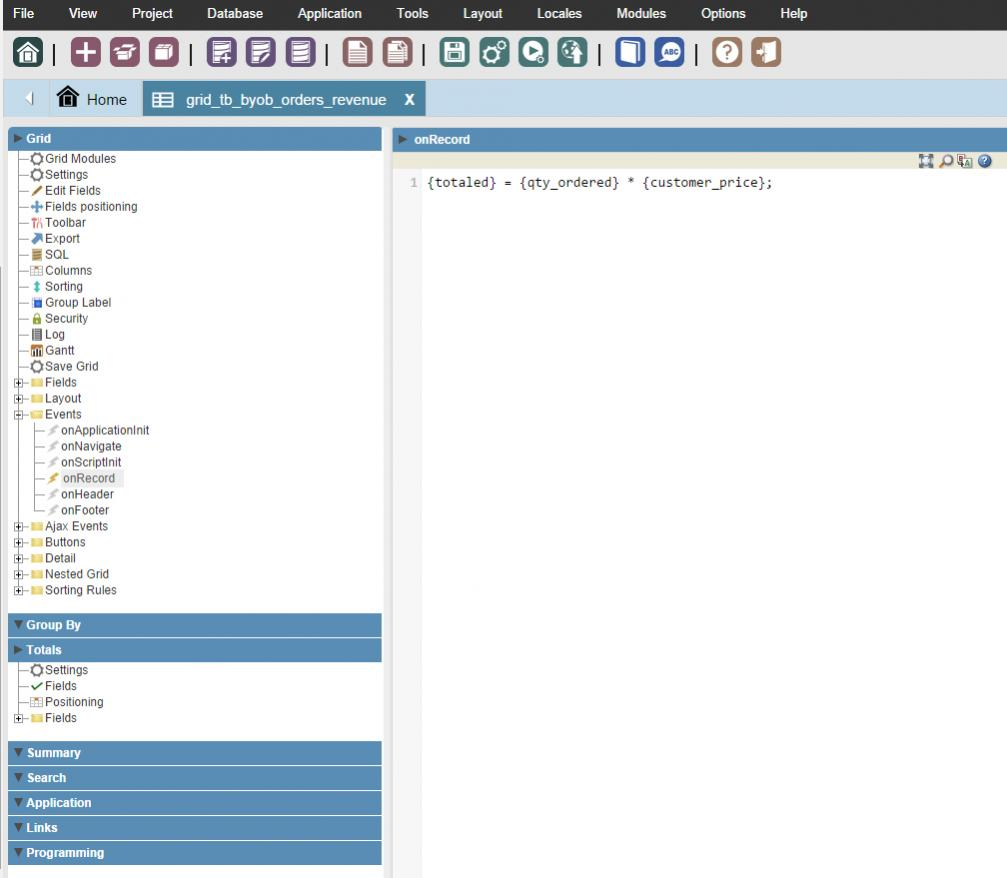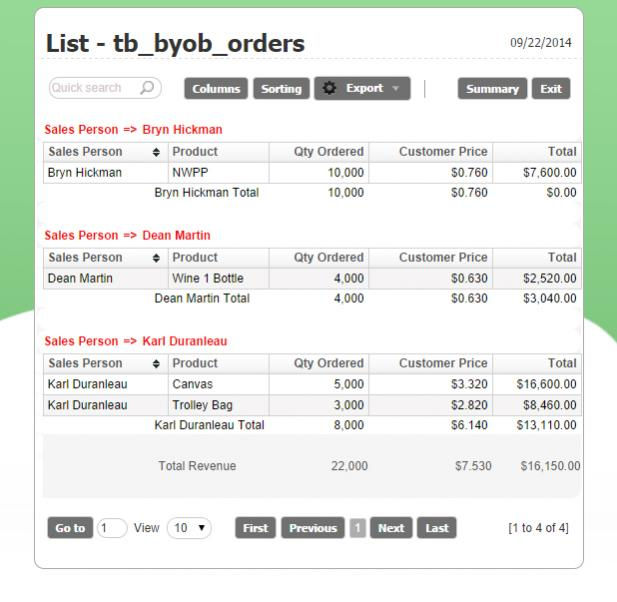# Totals on "Group by" column are adding up incorrectly?

Hello,

Has anyone seen this problem before ? (See Image)

“Sales Person”, “Product”, and “Qty Ordered” are coming from the orders table.

“Customer Price” is coming from the “Products Table” and Total is a calculated field.

Formula for {total} is… {total} = {qty_ordered} * {customer_price}

Thanks works fine but when I turn on totals for the “total” column in SC I get incorrect SUMS as shown in the image attached?

Anyone know why?hi instore

can you check the totals configuration, see if the formulas set there is correct, there is avarage, sum, max, min…etc. or perhaps you have some field is calculated… try totalizing one by one and see what happens

Take a look at the attached 3 screen grabs.

I don’t get it.

I get correct totals for all columns except the {totaled} column?

I have a simple formula to calculate the total.

Any thoughts on why this is incorrect?Let’s put it this way: It doesn’t work correctly on calculated fields.